# 5/8th Wave Antennas Why does the 5/8th have gain, and how much gain does it have?

A 5/8th antenna element, by itself, actually has no gain.
The 5/8th wave depends on radiation from a reflection or radiation from a second
source to create gain. We can see this by looking at some basic antennas some
distance above earth and away from ground planes or large reflective surfaces.

Here is a 5/8th wave vertical placed several wavelengths
above earth, with a counterpoise system too small to support low angle
reflections: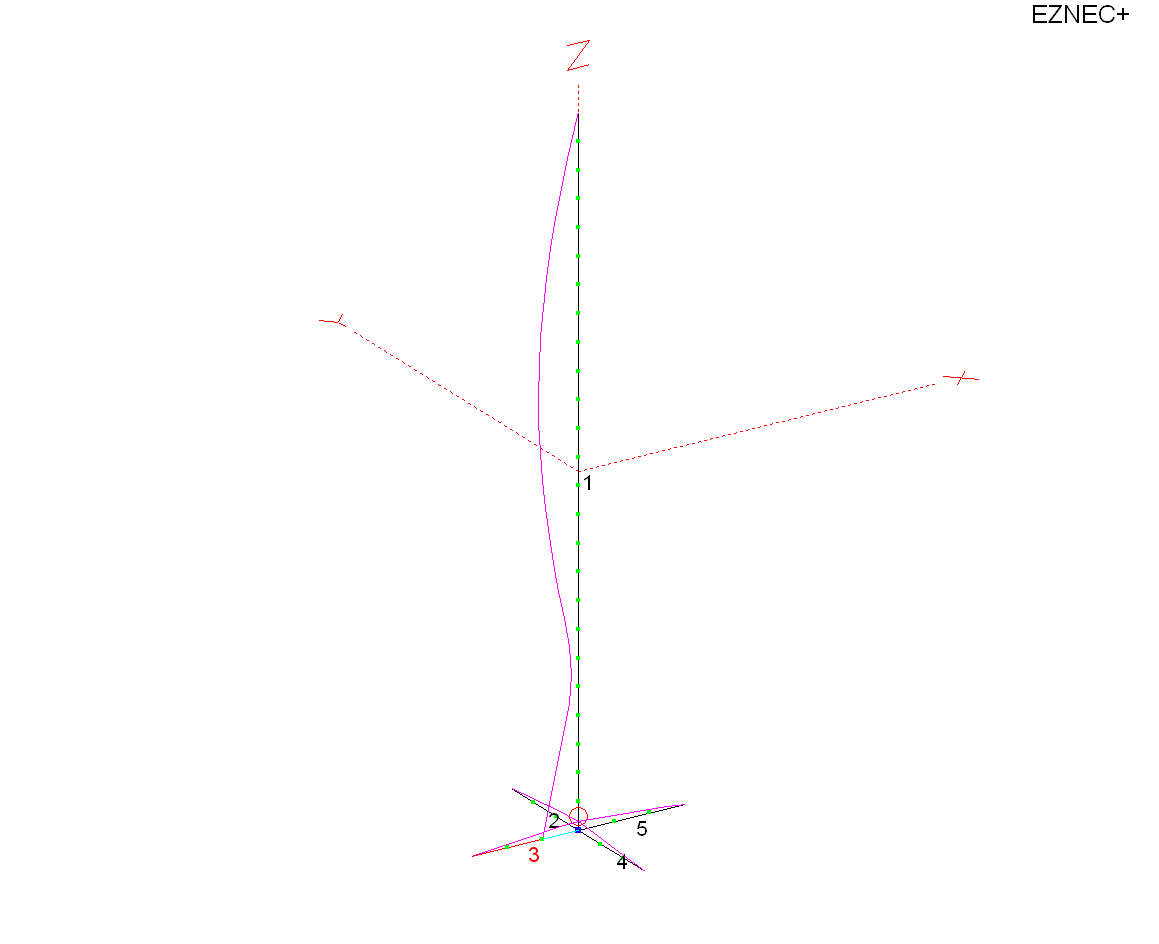This antenna has four short radials in the counterpoise,
and is .625 wavelengths long.

The pattern and gain is: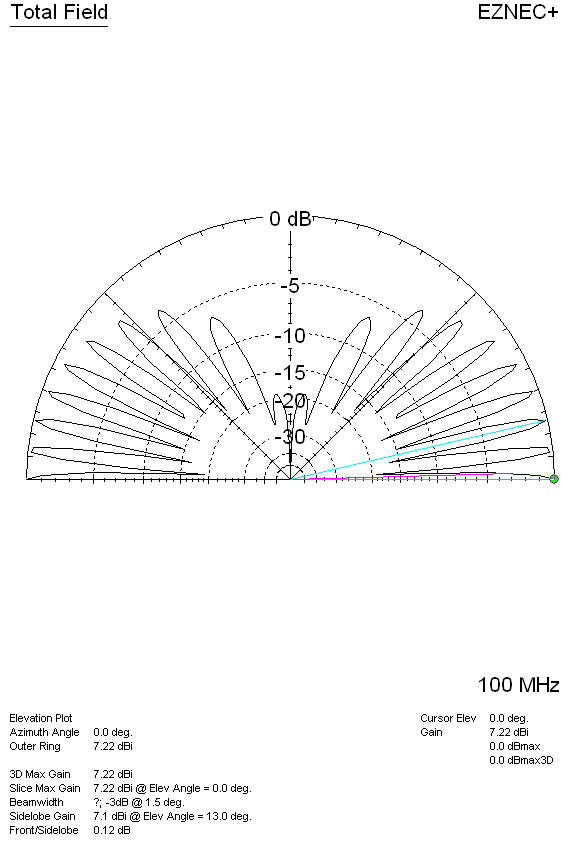We see the peak gain is 7.22 dBi, and it has lobes all
the way up to very high angles.

Now we can compare a 1/2 wave vertical dipole reference
antenna at the same base height: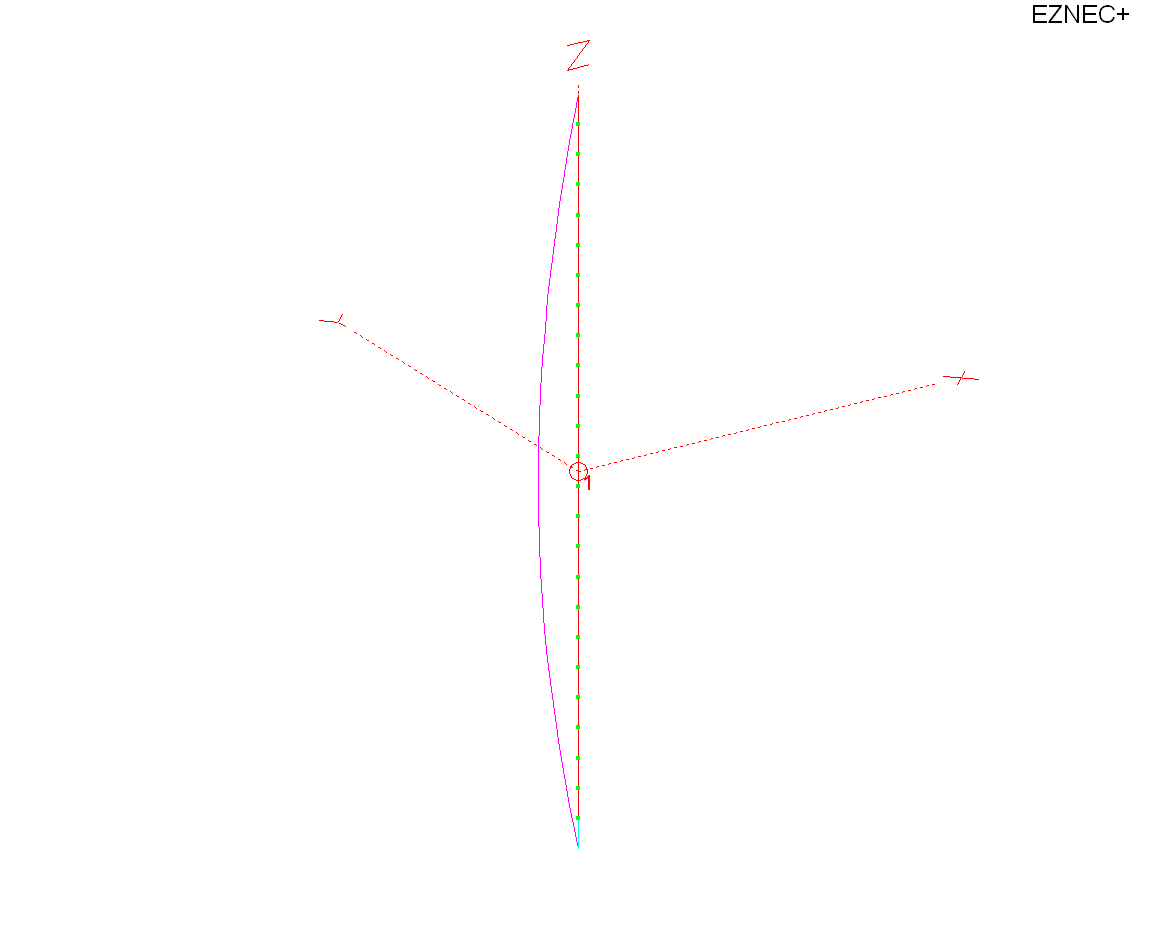Gain pattern plot of 1/2 wave antenna: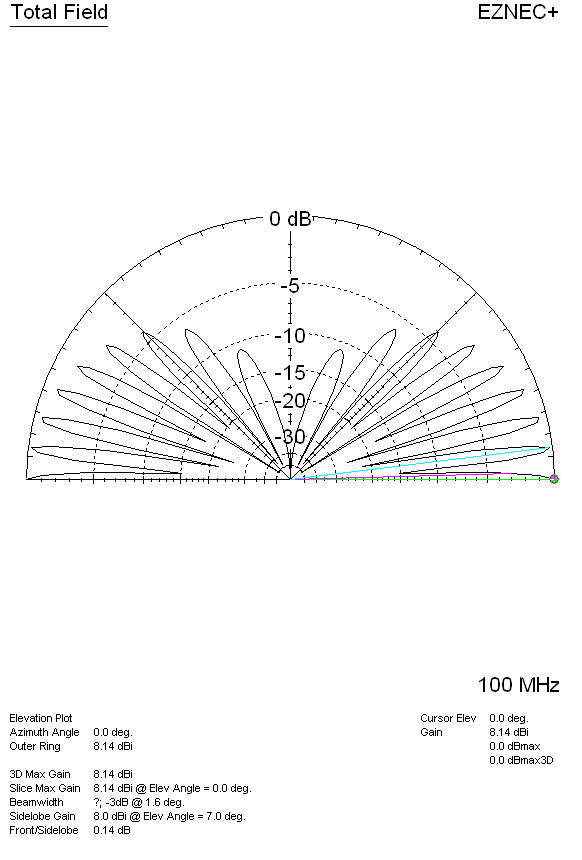We now see gain is 8.14 dBi maximum, and the gain along
earth at zero degrees is 8.14 dBi. This means the 5/8th wave antenna gain is
8.14-7.22 = 0.92 dB less than a dipole. The 5/8th wave has a net LOSS of signal
along the ground compared to a dipole at the same height, when both are remote
from earth or large ground planes.

Let’s look at a sloping-radial 1/4-wave antenna at the
same base height: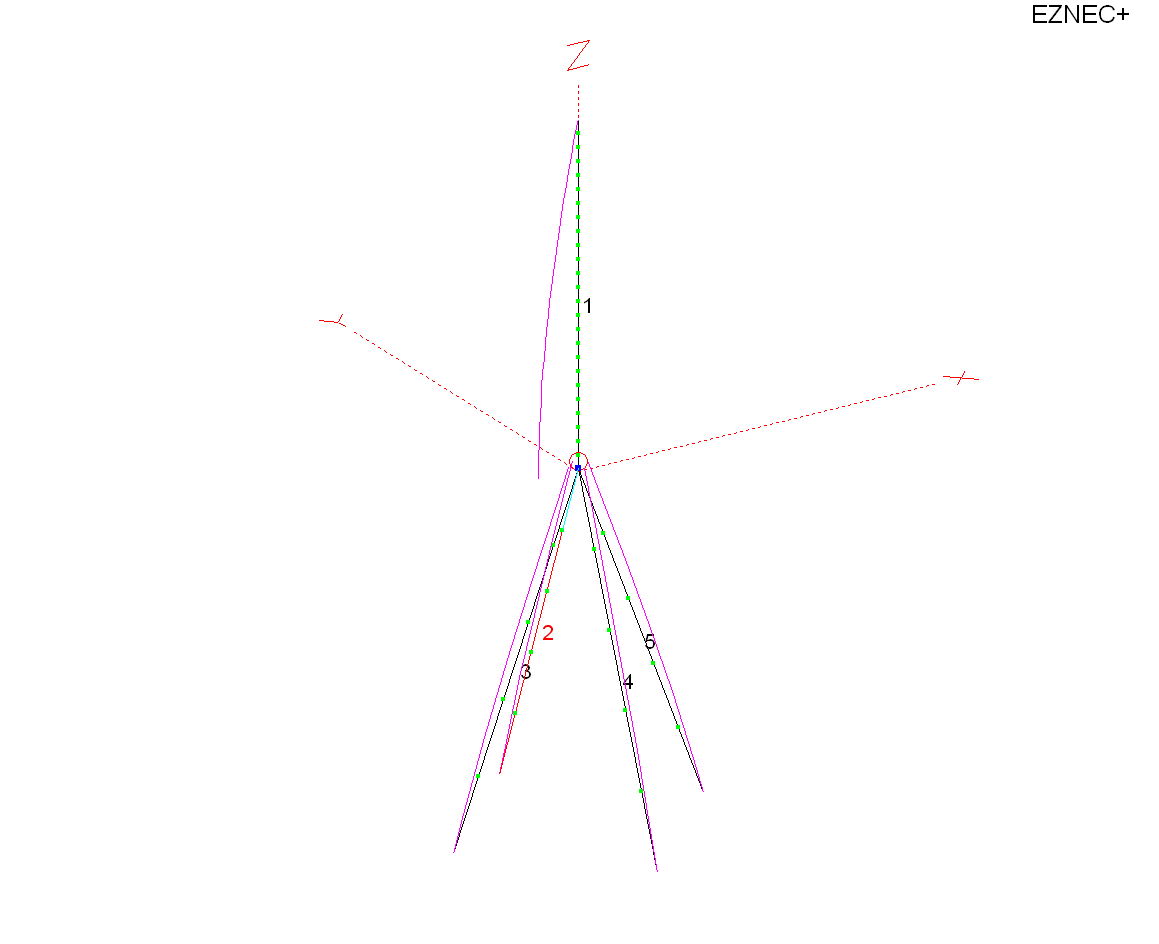We now see the pattern and gain is: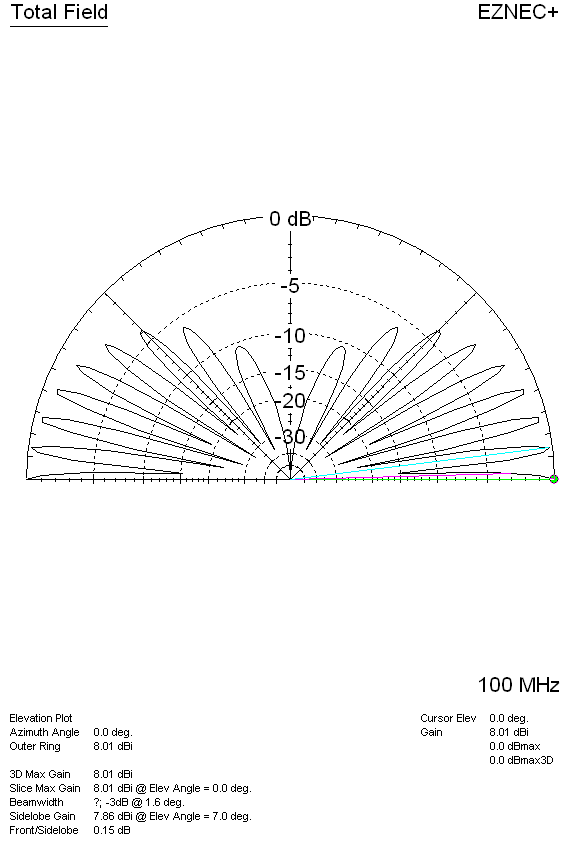Gain is now 8.01dBi. In direct comparison we
have:

 Antenna dBi dBd 1/2 wave 8.14 0 (reference dipole 4WL above earth) 1/4 WL ground plane sloped radials 8.01 -0.13 dBd 5/8th wave 7.22 -0.92 dBd

The 5/8th wave antenna has a net loss over a vertical
dipole when both antennas are located
far above earth. The 5/8 wave antenna also has a net loss over a λ/4
(quarter wave) ground plane with drooped radials. The 5/8λ antenna has a
net gain loss because it gets its gain from ground reflection some distance out
from the antenna base, and this antenna lacks the gain enhancement from a large reflecting ground plane
at base height, which means it lacks
the second source below the antenna base.

This is why professional commercial
antennas rarely use 5/8th wave elements, unless they are collinear above a
second source or ground mounted over highly conductive earth. For example, at
one time low frequency broadcast band stations used 5/8th wave verticals. The AM
broadcast use of 5/8λ verticals largely stopped because of increased
fading in fringe areas, and because the theoretical 3dB gain was rarely achieved
at most locations.

unobtainable gain as a characteristic of the 5/8th radiator, no matter where or
how it is installed. This created a 3dB gain  myth.
In fact, unless the 5/8λ antenna is compared to a 1/4 wave vertical, and
both the 5/8th wave and 1/4λ antennas are mounted directly over very
large, dense, highly conductive ground planes, or unless the system contains a second radiating source
that mirrors the 5/8λ element, they operate at a net loss.  This
article will walk through the basic antennas to explain how they actually work.

How Does a Vertical Omni Develop Elevation Pattern and
Gain?

A vertical, like any antenna, changes gain as the antenna
much loss will increase radiated field levels in other directions. Compressing
the pattern through the addition of nulls concentrates the applied power in a
smaller area of space, and that concentration of applied power is what we call “gain”.

Assuming loss remains about the same, a vertical
omnidirectional antenna develops gain through collinear effects. These collinear
vertical antenna, from ground reflections, or from both effects. Gain associated
with these effects closely follow the theoretical gain of a collinear antenna.

In the case of an antenna over highly conductive earth
(or a flat infinite ground plane), effects of ground plane reflection are analyzed
through use of an imaginary “image antenna”. The “image” mirrors the real
antenna’s current distribution and height, with the reversed image appearing an
equal distance below ground level. This is what causes the “collinear” earth
image effect.The graph
below, from Jasik’s Antenna Engineering Handbook, shows maximum possible
collinear theoretical gain, in this case caused by the image:Referring to the graph and image antenna drawings above,
we see a ground-mounted 1/2 wave vertical over infinite flat earth would have a
current maxima .25λ above earth, causing an “image” .25λ below
earth. This results in λ/2 (0.5 wave) spacing. If the reflection image is
perfect, the theoretical maximum possible gain would be about 1.8dB over an
antenna without any ground reflection gain. 1.8dB would be the gain of a λ/2
vertical or vertical dipole over a λ/4 vertical (quarter wave) with
current maxima at the ground level.

Elevating the current maxima above ground will increase
collinear spacing and increase gain. If we place the current maxima about 0.375λ
above earth, which is what a ground mounted 5/8λ element does, we see
theoretical image spacing is .75λ. Theoretical gain becomes 3dB over the
ground-mounted 1/4λ vertical case, and 1.2dB over the vertical dipole or
1/2λ (λ/2) ground mounted vertical case.

There is a slight loss of gain in the 5/8λ antenna
because a small section carries out-of-phase currents. These current are lower
than the main currents, and are less area, so they have significantly less
ampere-feet to cause radiation. The destructive interference at low angles is
less than the reinforcement gain, so the overall antenna has gain, albeit less
gain than theoretical. It works out over an infinite perfect ground plane a
lossless 5/8th wave antenna has about 2.8dB gain over a ground mounted half wave
vertical.

We will see models confirm the theory and, if both the
reference and 5/8th wave antennas are lossless, 5/8th wave gain can range from
just over 1 dB above a half-wave antenna in ideal cases, to a negative gain when
the antenna is moved away from the perfect infinite reflective flat plane.

The 3dB gain of a 5/8th wave is a case that can only
occur when something is wrong with the reference antenna, and the reference is a
ground mounted 1/4 wave vertical. The unpredictability and potential of signal
loss is why commercial two-way vendors, in the peak of industry times when
engineers were skilled and antenna test ranges were used, almost never used
5/8th wave elements. The 3dB gain myth is a Ham and CB radio myth.

Mobile Antennas

Mobile antennas are unique, in that the vehicle roof acts like a large
reflector to set up the image. This “reflection image” from the large roof and
earth surrounding the vehicle can give the 5/8th wave gain over a
1/4 wave whip, when conditions are right. A 5/8th
antenna in the air far above earth, however, is an entirely different situation.

A 5/8th
wave is claimed to
have 3dB gain over a
1/4 wl mobile antenna.
Let’s see if that is
true.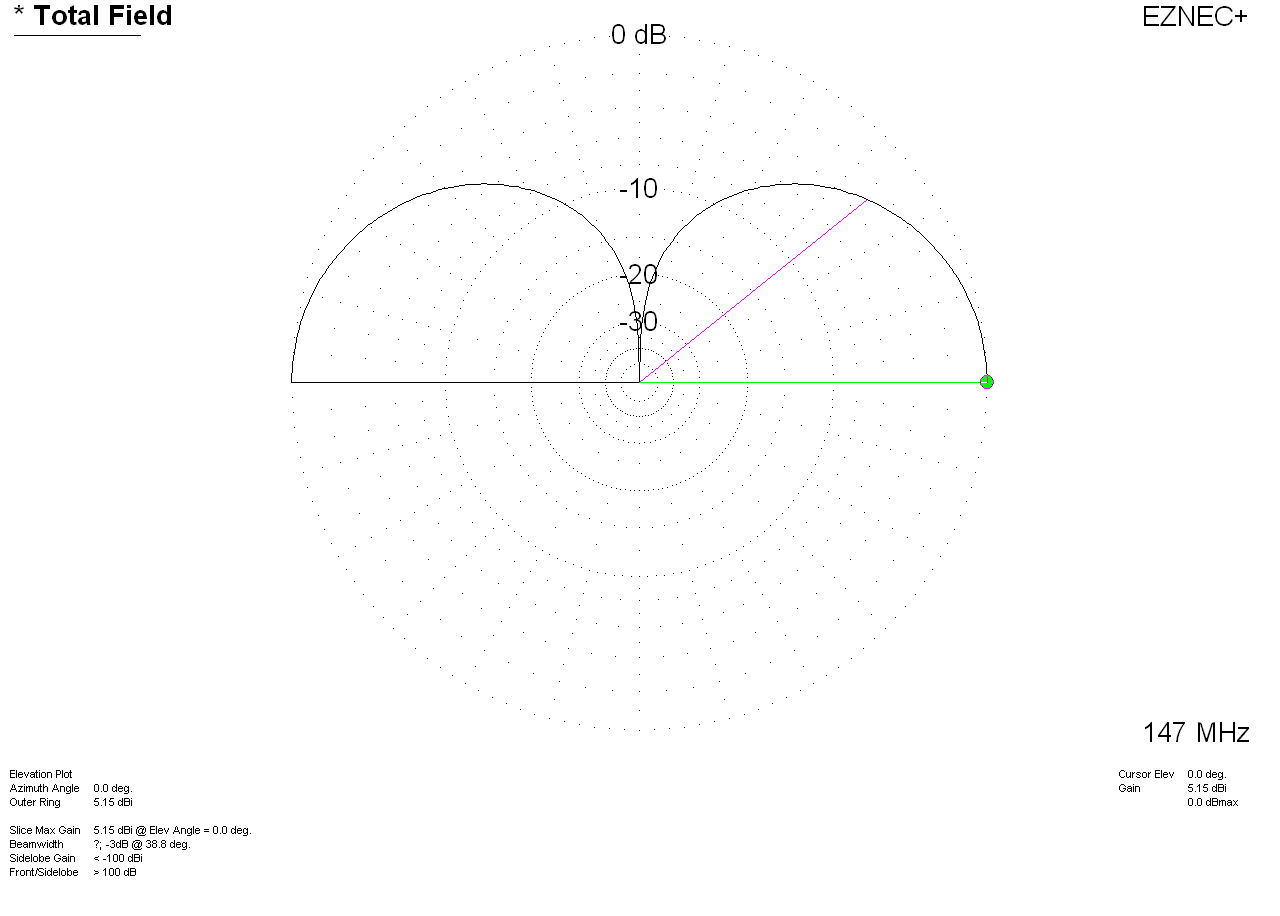Above….Pattern of a perfect
infinite ground 1/4
WL whip antenna. This antenna has 5.15 dBi gain. Let’s keep that in mind as a
reference gain.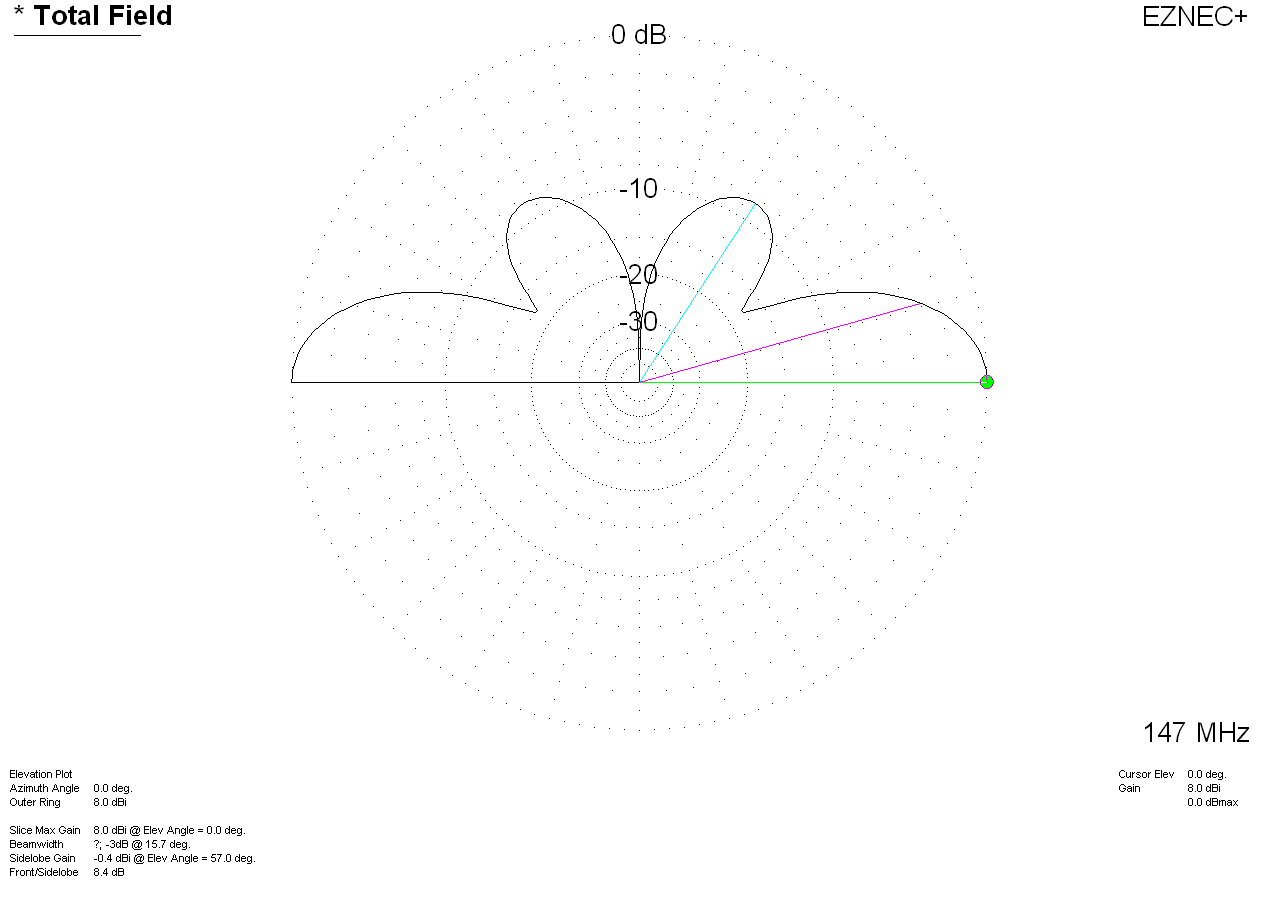Above pattern is a 5/8th wave over
perfect infinite
flat ground. We see the peak
gain is now 8dBi.
Gain over 1/4 wave
is 2.85db
assuming
NO matching system
losses.

The same infinite-ground plane antenna,
with a matching coil Q of
100, the 5/8th wave has 2.6dB
gain over the
reference 1/4
wave.

This is over an infinite size perfect ground extending to
infinity.

# Mounted on a roof to increase height above ground: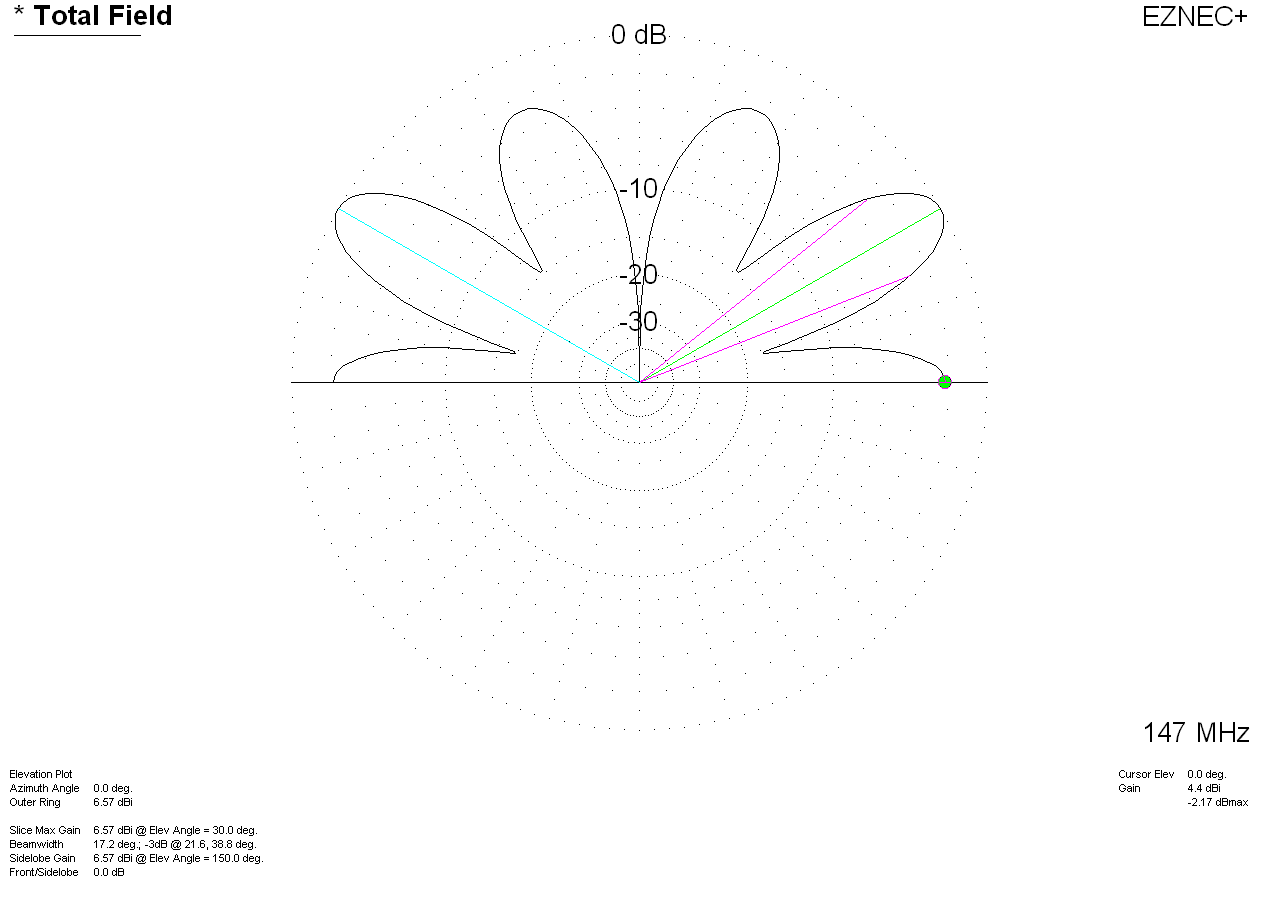This is a 1/4
wave antenna centered on a 1-meter radius plate five feet
high (that’s a big,
flat, and high
vehicle roof). It has 4.4dBi
gain at zero
degrees. Notice the
pattern change. This is over infinite perfect earth. Real earth would suppress
the lower angles further.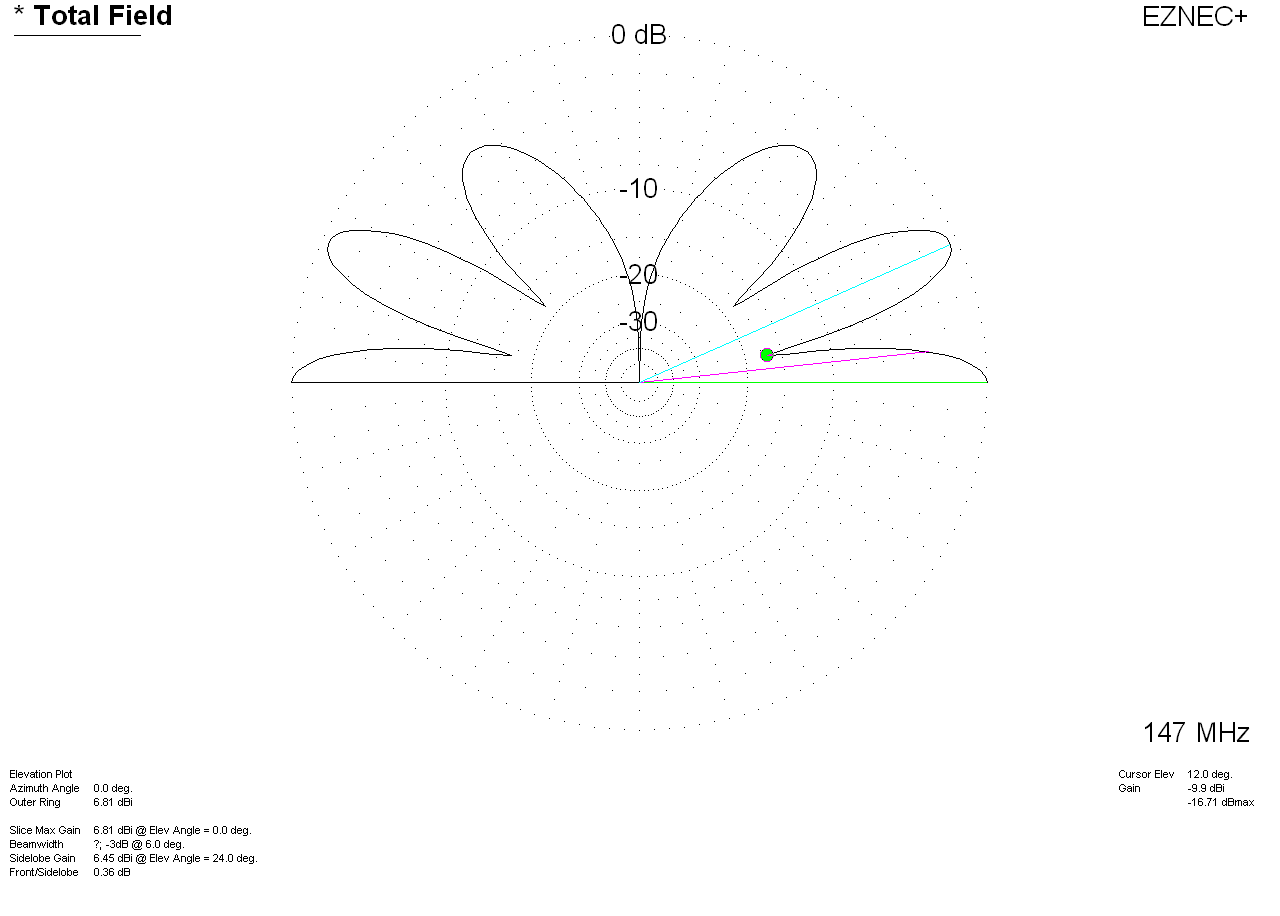This is a 5/8th
wave antenna centered on the same 1
high (again,  that is a large,
flat, high
vehicle roof). This “roof” is five
feet high over
perfect lossless
earth. This antenna has 6.81 dBi gain at
zero degrees. Notice
a similar
pattern change. This is because a
vertical antenna’s
pattern is largely
created by the
reflection from
earth around the
antenna.

The 5/8th
wave antenna gain with lossless matching would be
2.4dB better than
the 1/4 wave in this
case with the large roof as a flat ground plane.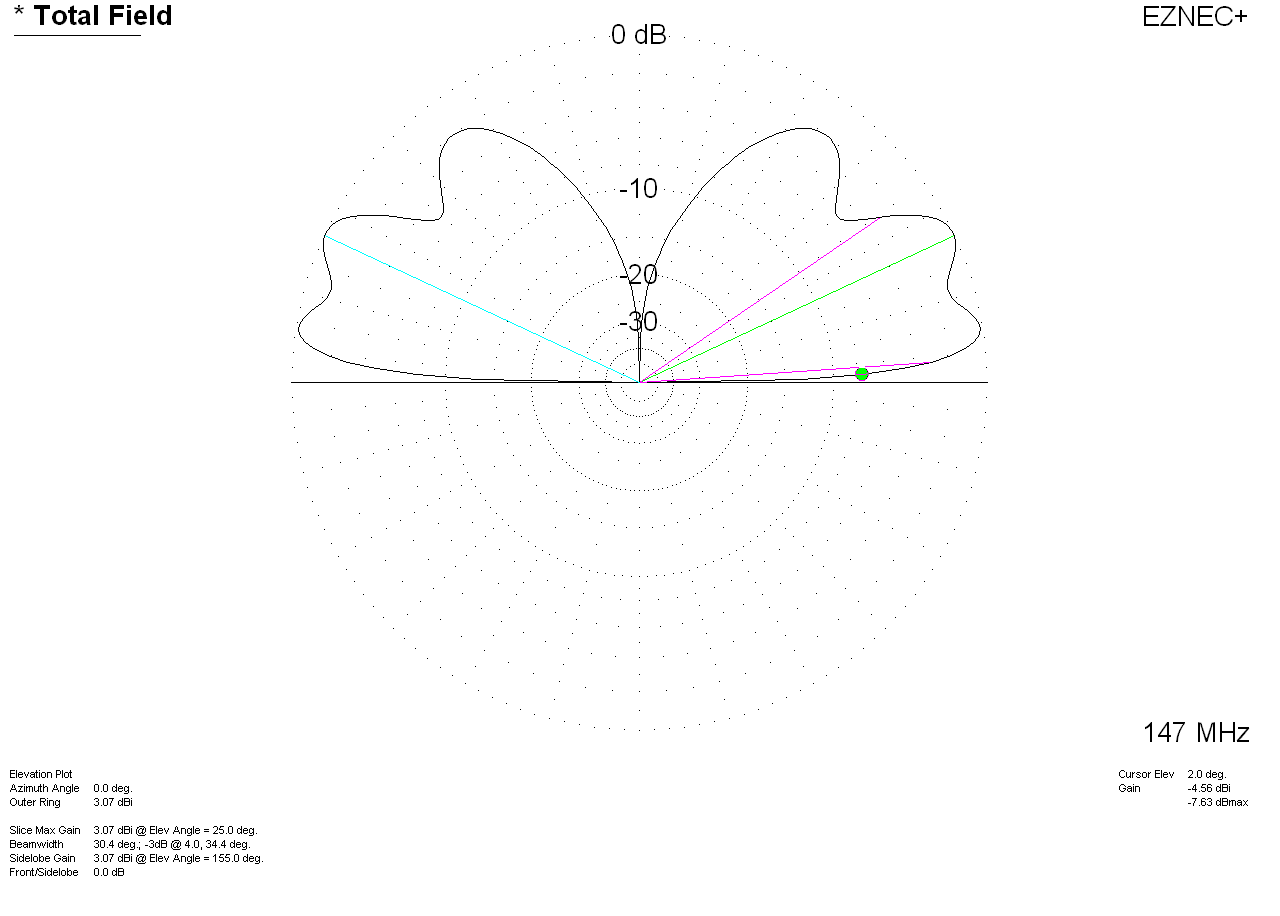The above is a 5/8th-wave
antenna centered on a large
flat roof over good
flat soil. It has
-4.56dBi gain at 2
degrees.

Maximum gain is
now only 3.07dBi.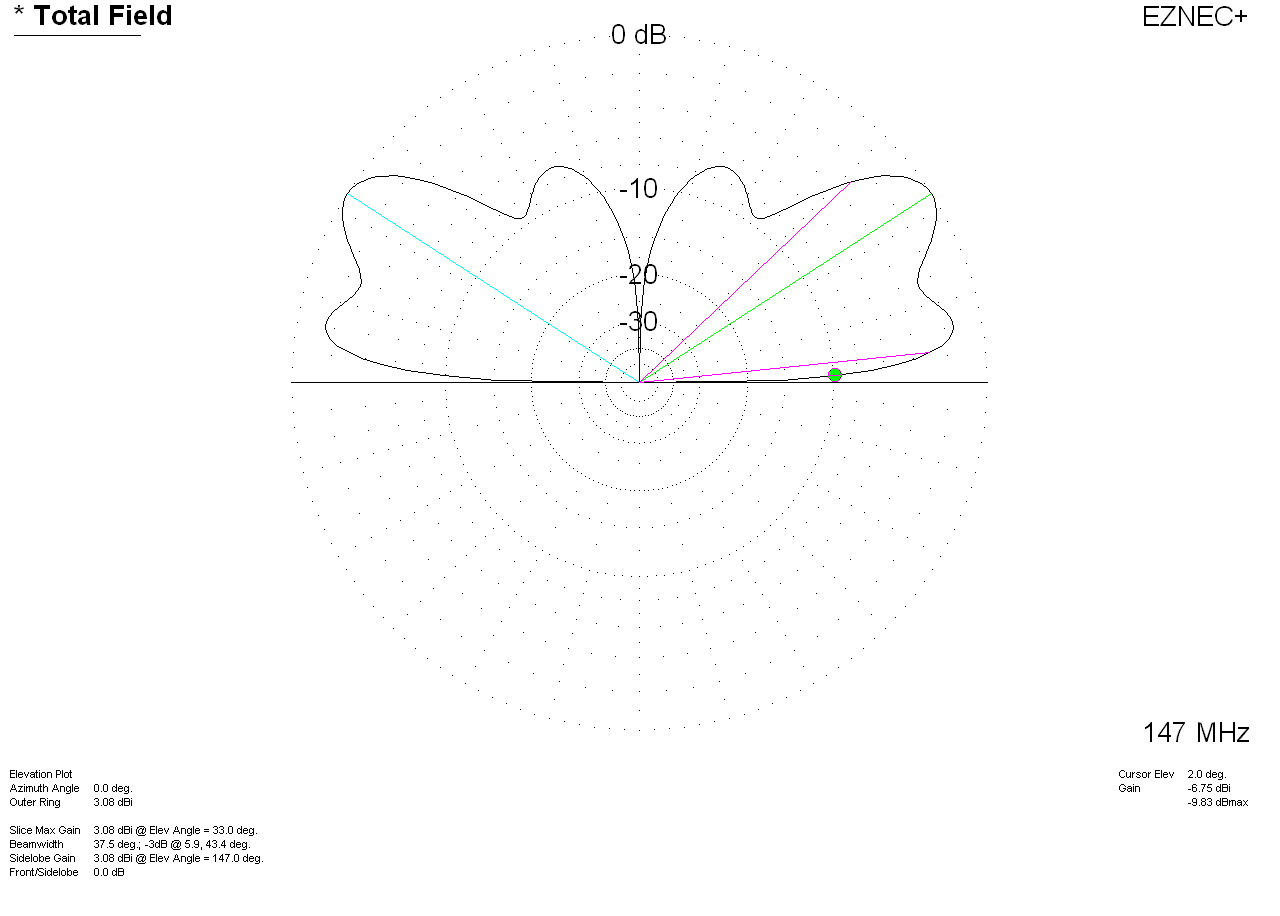At 2 degrees
takeoff the 1/4-wl antenna
over good soil with
perfectly flat
terrain has -6.75 dBi gain. Maximum
gain is now
3.08 dBi.

The gain of a
5/8th wave over a
1/4 wl antenna at 2
degrees is now 2.19
dB, but the peak
gain of the 5/8th wave is only .01dB
different than the
peak gain of a
1/4 wave antenna! The 2 degree gain of the 5/8th wave is mostly because of
increased height over lossy earth. The 2 degree gain of the 5/8th wave is not
because of antenna gain, but because of height change. It puts the current
further above earth.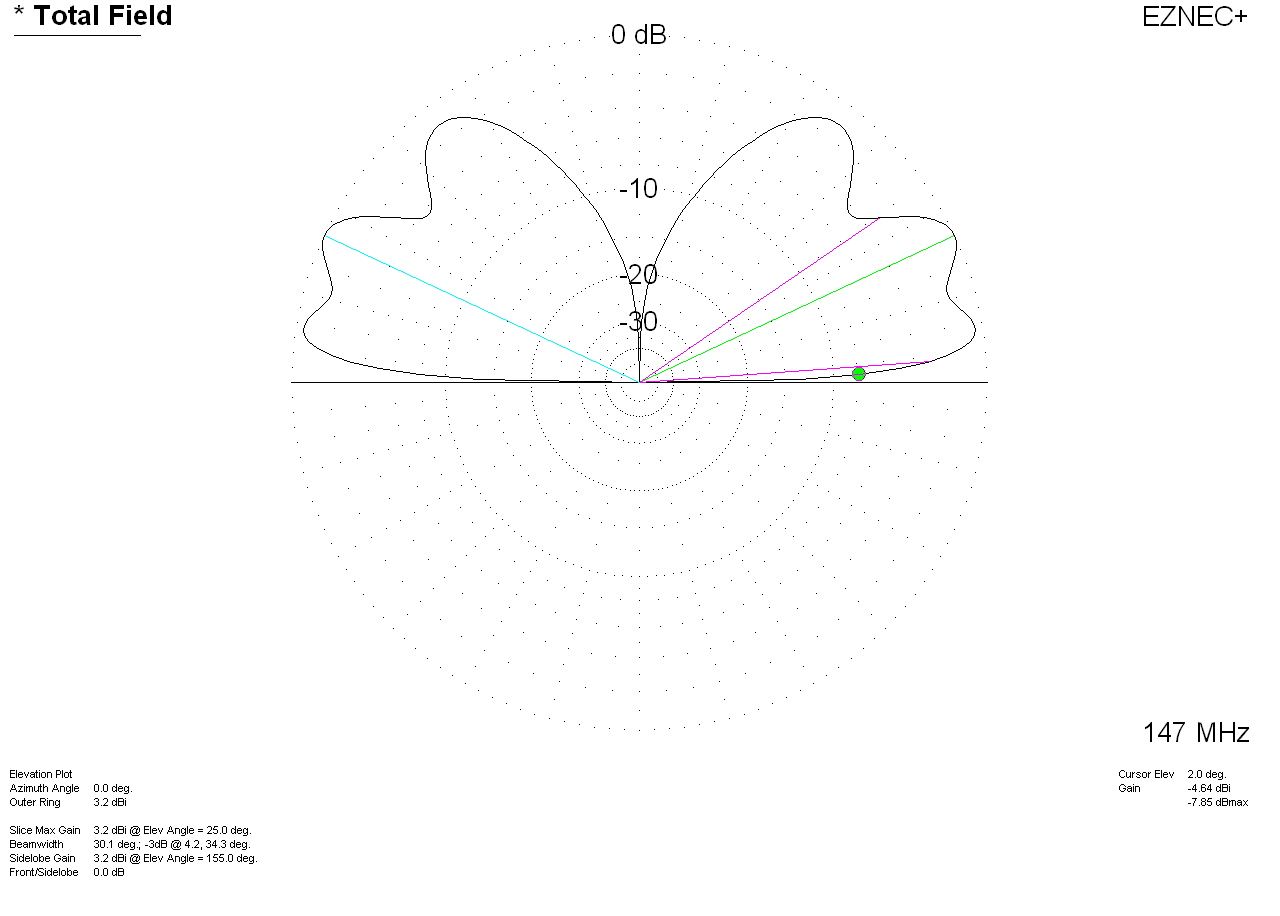With a normal
mid-size roof
contour 5/8th wave
gain is now
-4.64dBi at 2
degrees elevation.
Maximum gain is now
up slightly to
3.2dBi.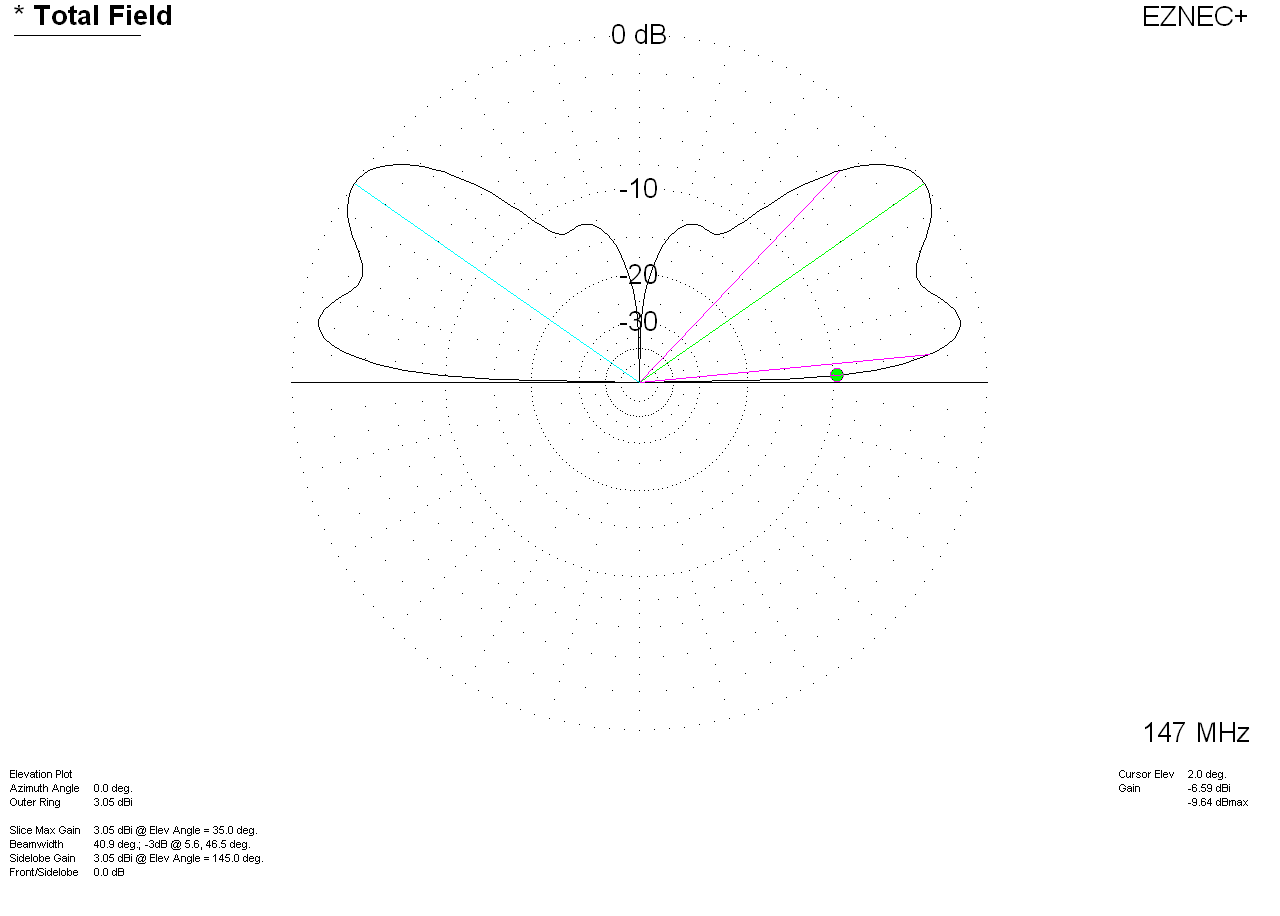With a normal
sloped roof shape and
mid-car size ground, the 1/4 wave has
-6.59dBi at 2
degrees TO.
Maximum gain is
3.05dBi.

5/8th
wave gain is now
1.95 dB over a 1/4
wave antenna at 2
degrees, but the
peak gain is only
.15dB better!

The smaller the
roof and/or the less
centered the
antenna, the less
5/8th wave. This occurs because 5/8th wave antennas develop gain from ground
reflections.

Lower height
roofs also have less
gain difference, and
shape of the roof
greatly affects
pattern and gain.

The 2.85dB
theoretical gain is
only for a perfect
coil antenna over
perfect infinite
flat ground plane.

In the real world
the actual gain
difference between a
1/4 wave antenna and
a 5/8th wave can be
anywhere from
no gain or a very slight loss up to a
maximum of 2dB gain over a 1/4 wave. The 5/8th wave is never really worse, explaining why people
have no problems using them. This is true for any repeater antenna height. The
primary advantage of a 5/8th wave in mobile operation is increased antenna
height, not from an actual gain increase.

The further from ground, the less advantage a 5/8th wave has.